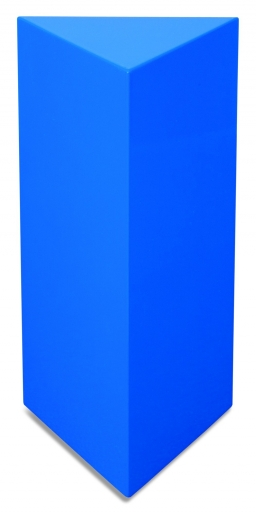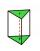# Triangular prism

The base of the perpendicular triangular prism is a rectangular triangle with a hypotenuse of 10 cm and one leg of 8 cm. The prism height is 75% of the perimeter of the base. Calculate the volume and surface of the prism.

V =  432 cm3
S =  480 cm2

### Step-by-step explanation:Did you find an error or inaccuracy? Feel free to write us. Thank you!Tips to related online calculators
Need help to calculate sum, simplify or multiply fractions? Try our fraction calculator.
Pythagorean theorem is the base for the right triangle calculator.
Tip: Our volume units converter will help you with the conversion of volume units.

#### You need to know the following knowledge to solve this word math problem:

We encourage you to watch this tutorial video on this math problem:

## Related math problems and questions:

• Triangular prismThe base of the perpendicular triangular prism is a right triangle with a leg length of 5 cm. The content area of the largest sidewall of its surface is 130 cm², and the height of the body is 10 cm. Calculate its volume.
• Perimeter and legsDetermine the perimeter of a right triangle if the length of one leg is 75% length of the second leg and its content area is 24 cm2.
• Vertical prismThe base of the vertical prism is a right triangle with leg a = 5 cm and a hypotenuse c = 13 cm. The height of the prism is equal to the circumference of the base. Calculate the surface area and volume of the prism
• Triangular prismThe perpendicular triangular prism is a right triangle with a 5 cm leg. The content of the prism's largest wall is 130 cm2, and the body height is 10 cm. Calculate the body volume.
• Triangular prismCalculate the volume and surface of the triangular prism ABCDEF with base of a isosceles triangle. Base's height is 16 cm, leg 10 cm, base height vc = 6 cm. The prism height is 9 cm.
• Triangular prism - regularThe regular triangular prism is 7 cm high. Its base is an equilateral triangle whose height is 3 cm. Calculate the surface and volume of this prism.
• Triangular prismThe base perpendicular triangular prism is a right triangle whose hypotenuse measures 5 cm and one cathetus 2 cm. Height of the prism is equal to 7/9 of the perimeter of the base. Calculate the surface area of prism.
• PrismThe base of a perpendicular triangular prism is a right triangle with legs 4.5 cm and 6 cm long. What is the surface of the prism, if its volume is 54 cubic centimeters?
• Base of prismThe base of the perpendicular prism is a rectangular triangle whose legs length are at a 3: 4 ratio. The height of the prism is 2cm smaller than the larger base leg. Determine the volume of the prism if its surface is 468 cm2.
• Triangular pyramidIt is given perpendicular regular triangular pyramid: base side a = 5 cm, height v = 8 cm, volume V = 28.8 cm3. What is it content (surface area)?
• PrismRight-angled prism, whose base is a right triangle with leg a = 3 cm and hypotenuse c = 13 cm, has the same volume as a cube with an edge length of 3 dm. a) Find the height of the prism b) Calculate the surface of the prism c) What percentage of the cube'
• 3sides prismThe base of vertical prism is an isosceles triangle whose base is 10 cm and the arm is 13 cm long. Prism height is three times the height of base triangle. Calculate the surface area of the prism.
• Triangular prismThe triangular prism has a base in the shape of a right triangle, the legs of which is 9 cm and 40 cm long. The height of the prism is 20 cm. What is its volume cm3? And the surface cm2?
• Right triangular prismWe have a cuboid with a base and dimensions of 12 cm and 5 cm and a height of 4 cm. The tablecloth is cut into two identical triangular prisms with right triangular bases. We painted the surface of the created prisms with color. Calculate the surface area
• Triangular pyramidCalculate the volume and surface area of a regular triangular pyramid with height equal to the base edge 10 cm length.Calculate the surface of a quadrilateral pyramid, which has a rectangular base with dimensions a = 8 cm, b = 6 cm and height H = 10 cm.The base of the prism is a regular hexagon consisting of six triangles with side a = 12 cm and height va = 10.4 cm. The prism height is 5 cm. Find the volume and surface of the prism.